Olympiad Test Level 2: Measurements - 2

# Olympiad Test Level 2: Measurements - 2

Test Description

## 10 Questions MCQ Test Math Olympiad for Class 2 | Olympiad Test Level 2: Measurements - 2

Olympiad Test Level 2: Measurements - 2 for Class 2 2022 is part of Math Olympiad for Class 2 preparation. The Olympiad Test Level 2: Measurements - 2 questions and answers have been prepared according to the Class 2 exam syllabus.The Olympiad Test Level 2: Measurements - 2 MCQs are made for Class 2 2022 Exam. Find important definitions, questions, notes, meanings, examples, exercises, MCQs and online tests for Olympiad Test Level 2: Measurements - 2 below.
Solutions of Olympiad Test Level 2: Measurements - 2 questions in English are available as part of our Math Olympiad for Class 2 for Class 2 & Olympiad Test Level 2: Measurements - 2 solutions in Hindi for Math Olympiad for Class 2 course. Download more important topics, notes, lectures and mock test series for Class 2 Exam by signing up for free. Attempt Olympiad Test Level 2: Measurements - 2 | 10 questions in 20 minutes | Mock test for Class 2 preparation | Free important questions MCQ to study Math Olympiad for Class 2 for Class 2 Exam | Download free PDF with solutions
 1 Crore+ students have signed up on EduRev. Have you?
Olympiad Test Level 2: Measurements - 2 - Question 1

### What is the weight of the apple?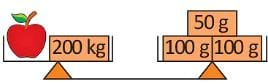Detailed Solution for Olympiad Test Level 2: Measurements - 2 - Question 1

Weight of an orange = 50 + 100 + 100 – 200 = 50 g

Olympiad Test Level 2: Measurements - 2 - Question 2

### How much should be subtracted from 65 kg 200 gram to get 43 kg 300 gram?

Detailed Solution for Olympiad Test Level 2: Measurements - 2 - Question 2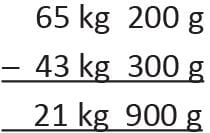Olympiad Test Level 2: Measurements - 2 - Question 3

### If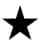is 70 kg, then the weight ofis ................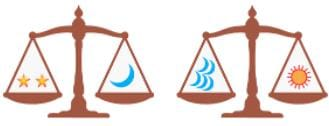Detailed Solution for Olympiad Test Level 2: Measurements - 2 - Question 3

Weight of sun = weight of 5 moon = 5 × 140 = 700 kg

Olympiad Test Level 2: Measurements - 2 - Question 4

Preeti is heavier than Suman. Nidhi is lighter than Ruchi. Suman is heavier than Ruchi. Who is heavier among them?

Detailed Solution for Olympiad Test Level 2: Measurements - 2 - Question 4

Preeti > Suman > Ruchi > Nidhi

Olympiad Test Level 2: Measurements - 2 - Question 5

Direction: Study the picture and answer the following questions: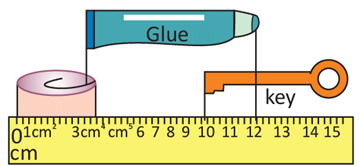What is the length of the glue tube?

Detailed Solution for Olympiad Test Level 2: Measurements - 2 - Question 5

12 cm – 3 cm = 9 cm

Olympiad Test Level 2: Measurements - 2 - Question 6

Direction: Study the picture and answer the following questions:What is the total length of the key, glue tube and paper clip?

Detailed Solution for Olympiad Test Level 2: Measurements - 2 - Question 6

3 cm + 9 cm + 5 cm = 17 cm

Olympiad Test Level 2: Measurements - 2 - Question 7

Saurabh is comparing weights of some objects. He wrote some sentences. Write true (T), false (F) for them.
(A) A book is lighter than pen.
(B) A pencil box is lighter than school bag.
(C) A scooter is lighter than truck.
(D) A football is heavier than a feather.

Olympiad Test Level 2: Measurements - 2 - Question 8

Directions: Read the table below and answer the following questions.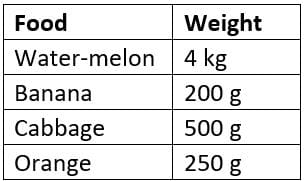Q. Which food is the heaviest?

Olympiad Test Level 2: Measurements - 2 - Question 9

Directions: Read the table below and answer the following questions.Q. Akshat bought 2 kg of a food. On purchasing which food he will get maximum number of that food?

Detailed Solution for Olympiad Test Level 2: Measurements - 2 - Question 9

Because weight of Banana is lighter.

Olympiad Test Level 2: Measurements - 2 - Question 10

Which combination a weights will keep both sides balanced on simple balance.

Detailed Solution for Olympiad Test Level 2: Measurements - 2 - Question 10

Half leg = 500 g. So, both sides of simple balance will remain balanced.

## Math Olympiad for Class 2

72 tests
 Use Code STAYHOME200 and get INR 200 additional OFF Use Coupon Code
Information about Olympiad Test Level 2: Measurements - 2 Page
In this test you can find the Exam questions for Olympiad Test Level 2: Measurements - 2 solved & explained in the simplest way possible. Besides giving Questions and answers for Olympiad Test Level 2: Measurements - 2, EduRev gives you an ample number of Online tests for practice

72 tests